# Taylor Series: Definition, Formula & Examples

Lesson Transcript
Instructor
Gerald Lemay

Gerald has taught engineering, math and science and has a doctorate in electrical engineering.

Expert Contributor
Simona Hodis

Simona received her PhD in Applied Mathematics in 2010 and is a college professor teaching undergraduate mathematics courses.

In mathematics, we sometimes gain insights by expressing functions in different ways. One of those ways is the Taylor series. In this lesson, we will define the Taylor series and use examples to demonstrate its use. Updated: 05/21/2020

## What Is the Taylor Series?

World politics can be complicated. Distant economies change dramatically. Far away populations move their centers. Sometimes, it's better to focus on what is happening locally. The same may be said for complicated mathematical expressions. By looking at the localized behavior of a function, we can often gain amazing insights. This is where the Taylor series is really useful. Simply put, the Taylor series is a representation of a function that can help us do mathematics. Uses of the Taylor series include analytic derivations and approximations of functions.

If we have a function which we can differentiate, then we can express that function as a Taylor series with the formula you're looking at on your screen right now.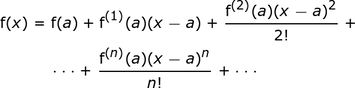Here are a few comments about notation and other particulars for this series. First of all, the derivative could be written as the formula you're now looking at on your screen: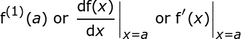This is telling us to differentiate with respect to x and then to substitute the x variable with the a variable. We have not completely eliminated the x variable; it still appears in the Taylor series formulation, only the x in the derivative gets replaced by a. There is also a factorial notation, which is shown by the exclamation point. It means, n! = n(n - 1)(n - 2) and so on. For example, 3! = 3(2)(1) = 6.

Although the Taylor series has an infinite number of terms, we often keep only a few terms. How many terms we keep is determined by knowing the convergence of the series. Basically, convergence means that as we include more and more terms, the sum of terms is not growing without bounds. A series may not converge. Analyzing how and if a series converges will tell us the values of x over which this series is valid.

In the examples used in this lesson, we will give this region of convergence information and not have to determine it ourselves. The a term in this series formula allows us to offset the series. We can then focus the series at a particular value on the x-axis. For this lesson, we will refer to the a term as the offset term.

An error occurred trying to load this video.

Try refreshing the page, or contact customer support.

Coming up next: How to Use the Second Derivative Test

### You're on a roll. Keep up the good work!

Replay
Your next lesson will play in 10 seconds
• 0:00 What Is the Taylor Series?
• 2:18 Making Sense of the…
• 5:03 Taylor Series…
• 6:16 Lesson Summary
Save Save

Want to watch this again later?

Timeline
Autoplay
Autoplay
Speed Speed

## Making Sense of the Offset Term

Let's determine the Taylor series for a particular function. Then we will plot a few terms to help us make sense of the a term. Consider the following function: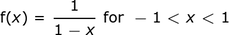From the Taylor series formula we see that we need derivatives of f(x). To make this easier, we write the following: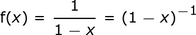Then, the first derivative is: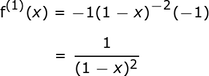And the second derivative of f2(x) equals: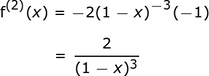Our Taylor series for this function, about the point a, is thus the following: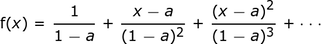for values of x between -1 and +1.

Let's say that we would like to match our curves at and near the point a = -0.5. In the following plot, the dark line is the desired function. The colored curve is the sum of only the first three terms of the Taylor series for a = -0.5. Note that f(x) is plotted as y in this and subsequent figures: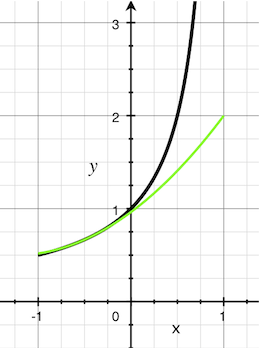Even with only three terms, we see that the curves match very well near x = -0.5, but not so well at x = 0. If we want a better match at x = 0, we let a = 0. The effect is to offset the series. Now our graph becomes what you're looking at on screen now: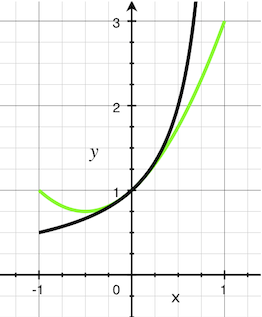To unlock this lesson you must be a Study.com Member.

## Taylor Series - Applications

### Review Topics

The Taylor series of a differentiable function, f(x) about an offset term a is given by the following series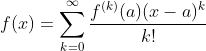A Taylor series is convergent if the sum of infinitely many terms is a finite number. To determine the convergence of a series, we usually apply a convergence test, like the ratio test.

### Taylor Series - Applications

Taylor series are used to approximate complex functions with polynomial functions, which are much easier to differentiate and integrate. The only disadvantage is that the function may be approximated with the Taylor series on a restrictive domain, the interval of convergence of the series.

For example, the exponential function is represented by the Taylor series around zero for any x, because the interval of convergence is the entire real axis,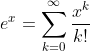To see how Taylor series is used to do algebra easier, we will evaluate the integral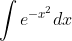with Taylor series, because it is challenging to obtain an antiderivative of the exponential function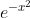### Application

1) Using the Taylor series of the exponential function, given above, write the Taylor series of2) Integrate the first three terms and the general term of the Taylor series obtained in 1).

3) Write the Taylor series around zero of the given integral.

### Solution

1)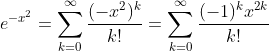2. An antiderivative of the first three terms is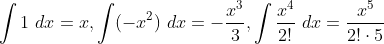An antiderivative of the general term is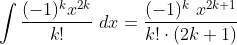3. The general antiderivative of the given exponential function is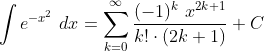### Register to view this lesson

Are you a student or a teacher?